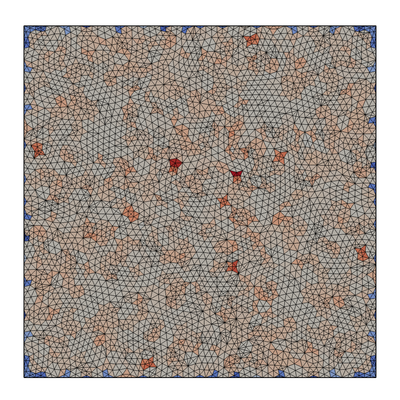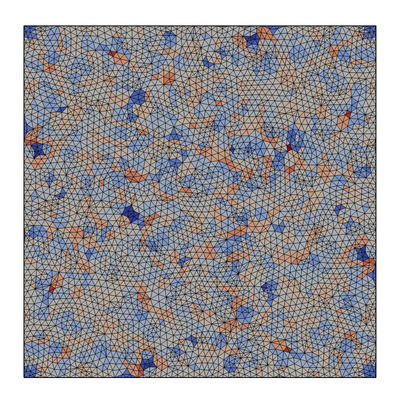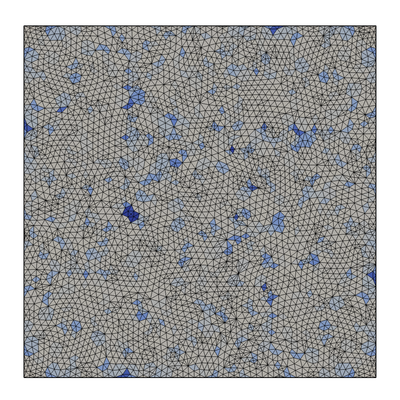## Introduction🔗

The test case comprises a 2-D section comprising extruded triangle cells. An initial field is assigned the mesh cell centre values such that the computed gradient should take the value of 1 in each co-ordinate direction. By changing the choice of gradient scheme we can observe how each performs.

## Case set-up🔗

• Gauss linear
• Gauss pointLinear
• leastSquares

Interpolation schemes under test:

### Boundary conditions🔗

Only the left and right domain boundaries are set to physical patch types; the remainder are set to the empty constraint to enforce the 2-D system.

### Mesh🔗

The mesh comprises extruded triangle cells with low non-orthogonality with average and maximum values of 7 and 26 deg, respectively.

### Scheme controls🔗

grad(Cc)        <scheme>


## Results🔗

The following images show the percentage error of the magnitude of the gradient of the cell centres, defined as: $$\textrm{error} = 100 \frac{\left|\grad(C_c)\right| - 2^{0.5}}{2^{0.5}}$$ The range is set to +/- 10% for all images.

### Unlimited🔗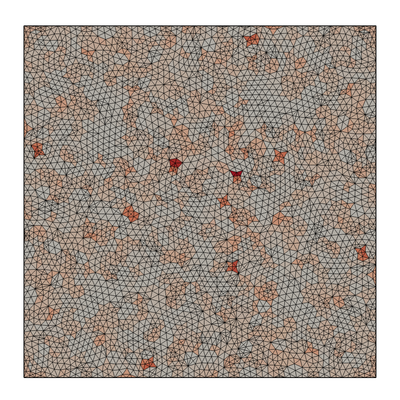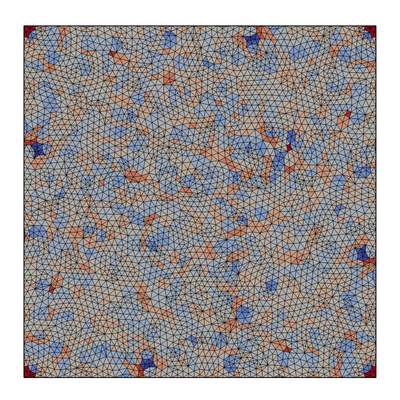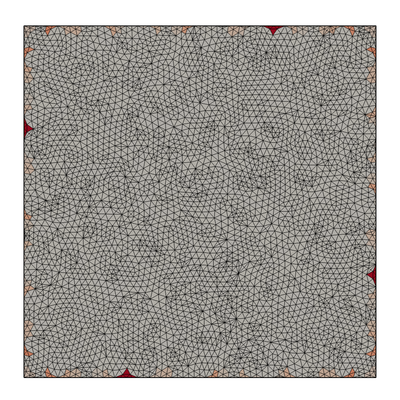### Cell limited🔗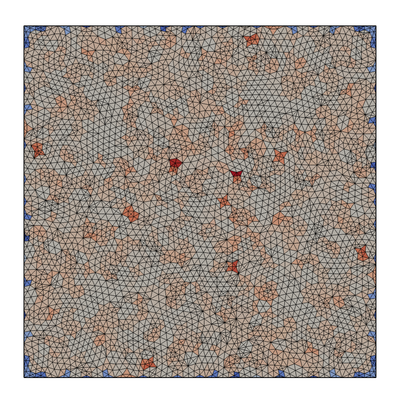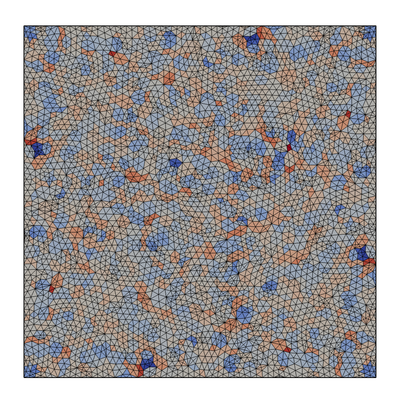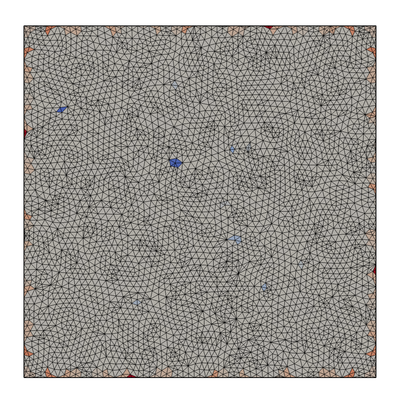### Face limited🔗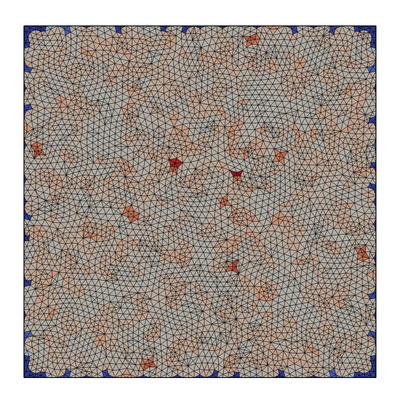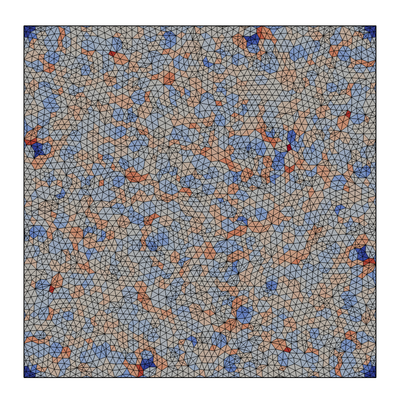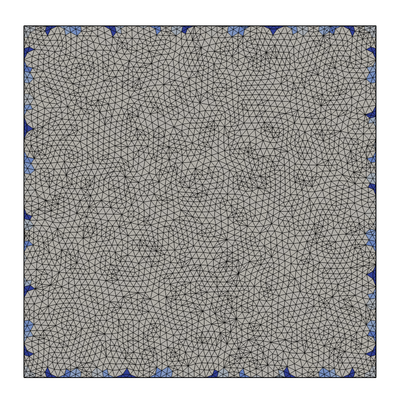### Multi-directional cell limited🔗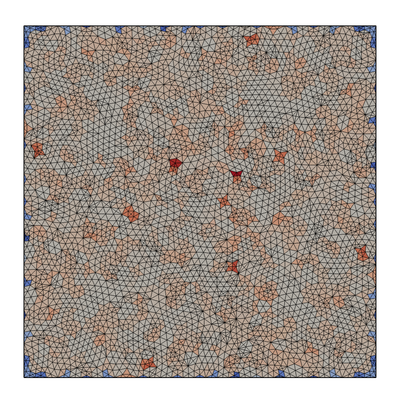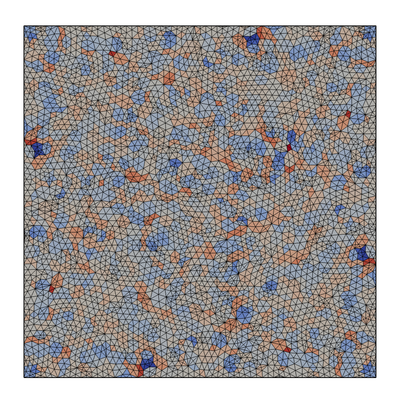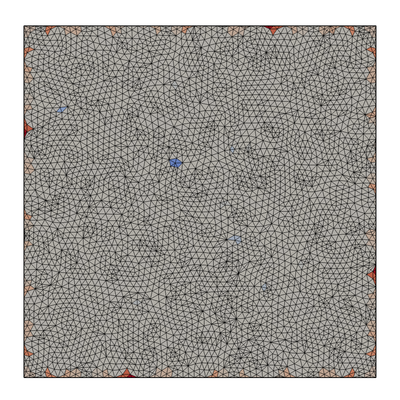### Multi-directional face limited🔗